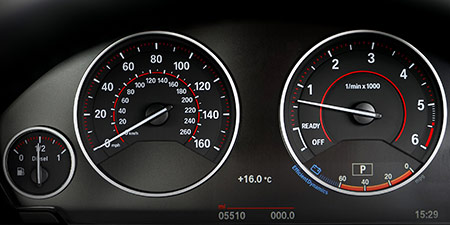# Speed of light to Kilometers per second (km/s)

Units of speed ﹣ Converter Speed of light to Kilometers per second

Here you can convert the Speed unit Speed of light into the unit Kilometers per second and vice versa you can convert Kilometers per second into Speed of light. By clicking the "Swap units" icon, you will always obtain the desired conversion in the calculation result. With the following calculator you can also calculate any other Speed unit.

## Info about "Speed of light"

Definition: If an object moves at the light speed for one second, it travels a distance of 299,792.458 km or about 300,000 km. This is the light speed that light travels in a vacuum.

This speed is also used to propagate radio signals, which is very important in space travel. For example, a signal from Mars takes about 3 to 20 minutes to reach Earth depending on its actual position. We notice a sunrise on earth about 8 minutes later because the light of the sun arrives to our eyes at that time.

## Info about "Kilometers per second"

Kilometer per second is part of the metric system and it is derived from the standard unit of speed, meter per second (m/s). This is because both meters and seconds are defined as the basic units for length and time respectively.

This unit is defined as follows: An object moving at "1 km/s" per second will cover one kilometer. "km/s" is approved to use in the international system of units (SI).

## Formula for the conversion of Speed of light to Kilometers per second (km/s) and vice versa

The calculation from Speed of light to Kilometers per second shall be made using the following conversion formula:

Conversion formula Speed of light to Kilometers per second

Determine the number of Kilometers per second from Speed of light

Speed of light × 299792.458

## Formula for the conversion of Kilometers per second (km/s) to Speed of light

The calculation from Kilometers per second to Speed of light shall be made using the following conversion formula:

Conversion formula Kilometers per second to Speed of light

Determine the number of Speed of light from Kilometers per second

Kilometers per second × 3.3356409519815E-6

## Overview table : How many Speed of light are in a Kilometers per second ?

Speed of light ⇒ Kilometers per second km/s
0.01   are  2 997.92458 km/s
0.02   are  5 995.84916 km/s
0.03   are  8 993.77374 km/s
0.04   are  11 991.69832 km/s
0.05   are  14 989.62290 km/s
0.06   are  17 987.54748 km/s
0.07   are  20 985.47206 km/s
0.08   are  23 983.39664 km/s
0.09   are  26 981.32122 km/s
0.10   are  29 979.24580 km/s
0.20   are  59 958.49160 km/s
0.30   are  89 937.73740 km/s
0.40   are  119 916.98320 km/s
0.50   are  149 896.22900 km/s
0.60   are  179 875.47480 km/s
0.70   are  209 854.72060 km/s
0.80   are  239 833.96640 km/s
0.90   are  269 813.21220 km/s
1   corresponds to  299 792.45800 km/s
2   are  599 584.91600 km/s
3   are  899 377.37400 km/s
4   are  1 199 169.83200 km/s
5   are  1 498 962.29000 km/s
6   are  1 798 754.74800 km/s
7   are  2 098 547.20600 km/s
8   are  2 398 339.66400 km/s
9   are  2 698 132.12200 km/s
10   are  2 997 924.58000 km/s
20   are  5 995 849.16000 km/s
30   are  8 993 773.74000 km/s
40   are  11 991 698.32000 km/s
50   are  14 989 622.90000 km/s
60   are  17 987 547.48000 km/s
70   are  20 985 472.06000 km/s
80   are  23 983 396.64000 km/s
90   are  26 981 321.22000 km/s
100   are  29 979 245.80000 km/s
200   are  59 958 491.60000 km/s
300   are  89 937 737.40000 km/s
400   are  119 916 983.20000 km/s
500   are  149 896 229 km/s
600   are  179 875 474.80000 km/s
700   are  209 854 720.60000 km/s
800   are  239 833 966.40000 km/s
900   are  269 813 212.20000 km/s
1 000   are  299 792 458 km/s

## Overview table : How many Kilometers per second are in a Speed of light ?

Kilometers per second km/s ⇒ Speed of light
0.01 km/s  are  0.0000000333
0.02 km/s  are  0.0000000667
0.03 km/s  are  0.0000001000
0.04 km/s  are  0.0000001334
0.05 km/s  are  0.0000001667
0.06 km/s  are  0.0000002001
0.07 km/s  are  0.0000002334
0.08 km/s  are  0.0000002668
0.09 km/s  are  0.0000003002
0.10 km/s  are  0.0000003335
0.20 km/s  are  0.0000006671
0.30 km/s  are  0.0000010006
0.40 km/s  are  0.0000013342
0.50 km/s  are  0.0000016678
0.60 km/s  are  0.0000020013
0.70 km/s  are  0.0000023349
0.80 km/s  are  0.0000026685
0.90 km/s  are  0.0000030020
1 km/s  corresponds to  0.0000033356
2 km/s  are  0.0000066712
3 km/s  are  0.0000100069
4 km/s  are  0.0000133425
5 km/s  are  0.0000166782
6 km/s  are  0.0000200138
7 km/s  are  0.0000233494
8 km/s  are  0.0000266851
9 km/s  are  0.0000300207
10 km/s  are  0.0000333564
20 km/s  are  0.0000667128
30 km/s  are  0.0001000692
40 km/s  are  0.0001334256
50 km/s  are  0.0001667820
60 km/s  are  0.0002001384
70 km/s  are  0.0002334948
80 km/s  are  0.0002668512
90 km/s  are  0.0003002076
100 km/s  are  0.0003335641
200 km/s  are  0.0006671281
300 km/s  are  0.0010006922
400 km/s  are  0.0013342563
500 km/s  are  0.0016678204
600 km/s  are  0.0020013845
700 km/s  are  0.0023349486
800 km/s  are  0.0026685127
900 km/s  are  0.0030020768
1 000 km/s  are  0.0033356409

## Source information

As source for the information in the 'Units of speed' category, we have used in particular:

## Last update on February 19, 2023

The pages of the 'Units of speed' category were last editorially reviewed by Stefan Banse on February 19, 2023. They all correspond to the current status.

### Previous changes on October 9, 2021

• November 9, 2020: Publication of the Speed converter
• Editorial revision of all texts in this category